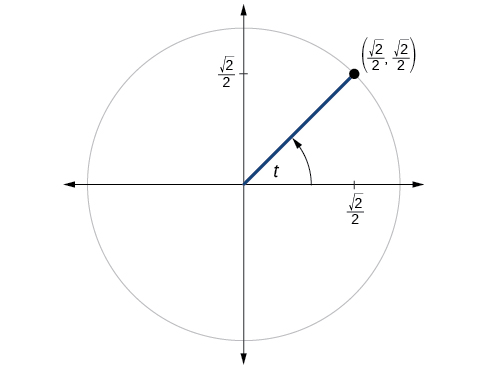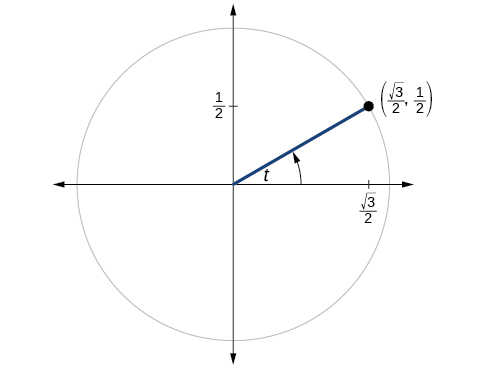# 7.4 The other trigonometric functions  (Page 7/14)

 Page 7 / 14

If $\mathrm{tan}\text{\hspace{0.17em}}t=\frac{12}{5},$ and $\text{\hspace{0.17em}}0\le t<\frac{\pi }{2},$ find $\text{\hspace{0.17em}}\mathrm{sin}\text{\hspace{0.17em}}t,\mathrm{cos}\text{\hspace{0.17em}}t,\mathrm{sec}\text{\hspace{0.17em}}t,\mathrm{csc}\text{\hspace{0.17em}}t,\text{and}\text{\hspace{0.17em}}\mathrm{cot}\text{\hspace{0.17em}}t.$

If $\text{\hspace{0.17em}}\mathrm{sin}\text{\hspace{0.17em}}t=\frac{\sqrt{3}}{2}\text{\hspace{0.17em}}$ and $\text{\hspace{0.17em}}\mathrm{cos}\text{\hspace{0.17em}}t=\frac{1}{2},$ find $\text{\hspace{0.17em}}\mathrm{sec}\text{\hspace{0.17em}}t,\mathrm{csc}\text{\hspace{0.17em}}t,\mathrm{tan}\text{\hspace{0.17em}}t,$ and $\text{\hspace{0.17em}}\mathrm{cot}\text{\hspace{0.17em}}t.$

$\mathrm{sec}t=2,$ $\mathrm{cot}t=\frac{\sqrt{3}}{3}$

If $\text{\hspace{0.17em}}\mathrm{sin}\text{\hspace{0.17em}}40°\approx 0.643\text{\hspace{0.17em}}$ and $\text{\hspace{0.17em}}\mathrm{cos}\text{\hspace{0.17em}}40°\approx 0.766,$ find $\text{\hspace{0.17em}}\text{sec}\text{\hspace{0.17em}}40°,\text{csc}\text{\hspace{0.17em}}40°,\text{tan}\text{\hspace{0.17em}}40°,$ and $\text{\hspace{0.17em}}\text{cot}\text{\hspace{0.17em}}40°.$

If $\text{\hspace{0.17em}}\text{sin}\text{\hspace{0.17em}}t=\frac{\sqrt{2}}{2},$ what is the $\text{\hspace{0.17em}}\text{sin}\left(-t\right)?$

$-\frac{\sqrt{2}}{2}$

If $\text{\hspace{0.17em}}\text{cos}\text{\hspace{0.17em}}t=\frac{1}{2},$ what is the $\text{\hspace{0.17em}}\text{cos}\left(-t\right)?$

If $\text{\hspace{0.17em}}\text{sec}\text{\hspace{0.17em}}t=3.1,$ what is the $\text{\hspace{0.17em}}\text{sec}\left(-t\right)?$

3.1

If $\text{\hspace{0.17em}}\text{csc}\text{\hspace{0.17em}}t=0.34,$ what is the $\text{\hspace{0.17em}}\text{csc}\left(-t\right)?$

If $\text{\hspace{0.17em}}\text{tan}\text{\hspace{0.17em}}t=-1.4,$ what is the $\text{\hspace{0.17em}}\text{tan}\left(-t\right)?$

1.4

If $\text{\hspace{0.17em}}\text{cot}\text{\hspace{0.17em}}t=9.23,$ what is the $\text{\hspace{0.17em}}\text{cot}\left(-t\right)?$

## Graphical

For the following exercises, use the angle in the unit circle to find the value of the each of the six trigonometric functions.$\mathrm{sin}t=\frac{\sqrt{2}}{2},\mathrm{cos}t=\frac{\sqrt{2}}{2},\mathrm{tan}t=1,\mathrm{cot}t=1,\mathrm{sec}t=\sqrt{2},\mathrm{csc}t=\sqrt{2}$$\mathrm{sin}t=-\frac{\sqrt{3}}{2},\mathrm{cos}t=-\frac{1}{2},\mathrm{tan}t=\sqrt{3},\mathrm{cot}t=\frac{\sqrt{3}}{3},\mathrm{sec}t=-2,\mathrm{csc}t=-\frac{2\sqrt{3}}{3}$

## Technology

For the following exercises, use a graphing calculator to evaluate to three decimal places.

$\mathrm{csc}\text{\hspace{0.17em}}\frac{5\pi }{9}$

$\mathrm{cot}\text{\hspace{0.17em}}\frac{4\pi }{7}$

–0.228

$\mathrm{sec}\text{\hspace{0.17em}}\frac{\pi }{10}$

$\mathrm{tan}\text{\hspace{0.17em}}\frac{5\pi }{8}$

–2.414

$\mathrm{sec}\text{\hspace{0.17em}}\frac{3\pi }{4}$

$\mathrm{csc}\text{\hspace{0.17em}}\frac{\pi }{4}$

1.414

$\text{tan}\text{\hspace{0.17em}}98°$

$\mathrm{cot}\text{\hspace{0.17em}}33°$

1.540

$\mathrm{cot}\text{\hspace{0.17em}}140°$

$\mathrm{sec}\text{\hspace{0.17em}}310°$

1.556

## Extensions

For the following exercises, use identities to evaluate the expression.

If $\text{\hspace{0.17em}}\mathrm{tan}\left(t\right)\approx 2.7,$ and $\text{\hspace{0.17em}}\mathrm{sin}\left(t\right)\approx 0.94,$ find $\text{\hspace{0.17em}}\mathrm{cos}\left(t\right).$

If $\text{\hspace{0.17em}}\mathrm{tan}\left(t\right)\approx 1.3,$ and $\text{\hspace{0.17em}}\mathrm{cos}\left(t\right)\approx 0.61,$ find $\text{\hspace{0.17em}}\mathrm{sin}\left(t\right).$

$\mathrm{sin}\left(t\right)\approx 0.79$

If $\text{\hspace{0.17em}}\mathrm{csc}\left(t\right)\approx 3.2,$ and $\text{\hspace{0.17em}}\mathrm{cos}\left(t\right)\approx 0.95,$ find $\text{\hspace{0.17em}}\mathrm{tan}\left(t\right).$

If $\text{\hspace{0.17em}}\mathrm{cot}\left(t\right)\approx 0.58,$ and $\text{\hspace{0.17em}}\mathrm{cos}\left(t\right)\approx 0.5,$ find $\text{\hspace{0.17em}}\mathrm{csc}\left(t\right).$

$\mathrm{csc}t\approx 1.16$

Determine whether the function $\text{\hspace{0.17em}}f\left(x\right)=2\mathrm{sin}x\text{\hspace{0.17em}}\mathrm{cos}\text{\hspace{0.17em}}x\text{\hspace{0.17em}}$ is even, odd, or neither.

Determine whether the function $\text{\hspace{0.17em}}f\left(x\right)=3{\mathrm{sin}}^{2}x\text{\hspace{0.17em}}\mathrm{cos}\text{\hspace{0.17em}}x+\mathrm{sec}\text{\hspace{0.17em}}x\text{\hspace{0.17em}}$ is even, odd, or neither.

even

Determine whether the function $\text{\hspace{0.17em}}f\left(x\right)=\mathrm{sin}\text{\hspace{0.17em}}x-2{\mathrm{cos}}^{2}x\text{\hspace{0.17em}}$ is even, odd, or neither.

Determine whether the function $\text{\hspace{0.17em}}f\left(x\right)={\mathrm{csc}}^{2}x+\mathrm{sec}\text{\hspace{0.17em}}x\text{\hspace{0.17em}}$ is even, odd, or neither.

even

For the following exercises, use identities to simplify the expression.

$\mathrm{csc}\text{\hspace{0.17em}}t\text{\hspace{0.17em}}\mathrm{tan}\text{\hspace{0.17em}}t$

$\frac{\mathrm{sec}\text{\hspace{0.17em}}t}{\mathrm{csc}\text{\hspace{0.17em}}t}$

$\frac{\mathrm{sin}\text{\hspace{0.17em}}t}{\mathrm{cos}\text{\hspace{0.17em}}t}=\mathrm{tan}\text{\hspace{0.17em}}t$

## Real-world applications

The amount of sunlight in a certain city can be modeled by the function $\text{\hspace{0.17em}}h=15\mathrm{cos}\left(\frac{1}{600}d\right),$ where $\text{\hspace{0.17em}}h\text{\hspace{0.17em}}$ represents the hours of sunlight, and $\text{\hspace{0.17em}}d\text{\hspace{0.17em}}$ is the day of the year. Use the equation to find how many hours of sunlight there are on February 10, the 42 nd day of the year. State the period of the function.

The amount of sunlight in a certain city can be modeled by the function $\text{\hspace{0.17em}}h=16\mathrm{cos}\left(\frac{1}{500}d\right),$ where $\text{\hspace{0.17em}}h\text{\hspace{0.17em}}$ represents the hours of sunlight, and $\text{\hspace{0.17em}}d\text{\hspace{0.17em}}$ is the day of the year. Use the equation to find how many hours of sunlight there are on September 24, the 267th day of the year. State the period of the function.

13.77 hours, period: $\text{\hspace{0.17em}}1000\pi$

The equation $\text{\hspace{0.17em}}P=20\mathrm{sin}\left(2\pi t\right)+100\text{\hspace{0.17em}}$ models the blood pressure, $\text{\hspace{0.17em}}P,$ where $\text{\hspace{0.17em}}t\text{\hspace{0.17em}}$ represents time in seconds. (a) Find the blood pressure after 15 seconds. (b) What are the maximum and minimum blood pressures?

The height of a piston, $\text{\hspace{0.17em}}h,$ in inches, can be modeled by the equation $\text{\hspace{0.17em}}y=2\mathrm{cos}\text{\hspace{0.17em}}x+6,$ where $\text{\hspace{0.17em}}x\text{\hspace{0.17em}}$ represents the crank angle. Find the height of the piston when the crank angle is $\text{\hspace{0.17em}}55°.$

7.73 inches

The height of a piston, $\text{\hspace{0.17em}}h,$ in inches, can be modeled by the equation $\text{\hspace{0.17em}}y=2\mathrm{cos}\text{\hspace{0.17em}}x+5,$ where $\text{\hspace{0.17em}}x\text{\hspace{0.17em}}$ represents the crank angle. Find the height of the piston when the crank angle is $\text{\hspace{0.17em}}55°.$

## Angles

For the following exercises, convert the angle measures to degrees.

$45°$

$-\frac{5\pi }{3}$

For the following exercises, convert the angle measures to radians.

$-210°$

$-\frac{7\pi }{6}$

$180°$

Find the length of an arc in a circle of radius 7 meters subtended by the central angle of $\text{\hspace{0.17em}}85°.$

10.385 meters

By the definition, is such that 0!=1.why?
(1+cosA+IsinA)(1+cosB+isinB)/(cos@+isin@)(cos$+isin$)
hatdog
Mark
how we can draw three triangles of distinctly different shapes. All the angles will be cutt off each triangle and placed side by side with vertices touching
bsc F. y algebra and trigonometry pepper 2
given that x= 3/5 find sin 3x
4
DB
remove any signs and collect terms of -2(8a-3b-c)
-16a+6b+2c
Will
Joeval
(x2-2x+8)-4(x2-3x+5)
sorry
Miranda
x²-2x+9-4x²+12x-20 -3x²+10x+11
Miranda
x²-2x+9-4x²+12x-20 -3x²+10x+11
Miranda
(X2-2X+8)-4(X2-3X+5)=0 ?
master
The anwser is imaginary number if you want to know The anwser of the expression you must arrange The expression and use quadratic formula To find the answer
master
The anwser is imaginary number if you want to know The anwser of the expression you must arrange The expression and use quadratic formula To find the answer
master
Y
master
master
Soo sorry (5±Root11* i)/3
master
Mukhtar
2x²-6x+1=0
Ife
explain and give four example of hyperbolic function
What is the correct rational algebraic expression of the given "a fraction whose denominator is 10 more than the numerator y?
y/y+10
Mr
Find nth derivative of eax sin (bx + c).
Find area common to the parabola y2 = 4ax and x2 = 4ay.
Anurag
y2=4ax= y=4ax/2. y=2ax
akash
A rectangular garden is 25ft wide. if its area is 1125ft, what is the length of the garden
to find the length I divide the area by the wide wich means 1125ft/25ft=45
Miranda
thanks
Jhovie
What do you call a relation where each element in the domain is related to only one value in the range by some rules?
A banana.
Yaona
a function
Daniel
a function
emmanuel
given 4cot thither +3=0and 0°<thither <180° use a sketch to determine the value of the following a)cos thither
what are you up to?
nothing up todat yet
Miranda
hi
jai
hello
jai
Miranda Drice
jai
aap konsi country se ho
jai
which language is that
Miranda
I am living in india
jai
good
Miranda
what is the formula for calculating algebraic
I think the formula for calculating algebraic is the statement of the equality of two expression stimulate by a set of addition, multiplication, soustraction, division, raising to a power and extraction of Root. U believe by having those in the equation you will be in measure to calculate it
Miranda

#### Get Jobilize Job Search Mobile App in your pocket Now!ByByBy OpenStaxBy Janet ForresterBy OpenStaxBy OpenStaxBy Stephen VoronBy OpenStaxBy Brooke DelaneyBy Joli JuliannaBy Jessica CollettBy Briana Knowlton